Quanta and Waves Flashcards Preview

Linlithgow Academy Advanced Higher Physics > Quanta and Waves > Flashcards

Flashcards in Quanta and Waves Deck (61):
1

Considering the relationship shown for velocity in SHM, describe the conditions when the velocity is at a maximum and at a minimum.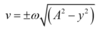2

3

Classical theory suggested that as an object becomes hotter, the irradiance of short wavelength radiation would increase dramatically. In fact, experiements show this irradiance is very small.What is this effect known as, and why does it happen?.4

The direction of the force exerted on a negatively charged particle entering a magnetic field perpendicularly can be determined using the 'right hand rule'. Describe the use of the 'right hand rule' by determining which 'finger' represents which quantity.5

6

7

8

9

10

Explain the production of 'cosmic air showers' when cosmic rays enter the atmosphere.

The cosmic ray strikes a nucleus creating secondary particles (usually hadrons such as the pion) which in turn collide with other particles and so on creating a 'shower' of particles which are detectable a ground level (such as muons, positrons and neutrinos).'Cosmic air showers' provided the very first evidence for sub nuclear particles and the quark model of matter.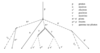11

12

13

14

A stationary wave is formed in a piece of elastic string under tension, created by a vibration generator set to 250Hz as shown below.The distance between adjacent nodes is 0.15m Determine the wavelength of this wave. Determine the speed of this wave.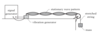15

The magnitude of the force on a charged particle entering a magnetic field can be determined using the relationshipF = qvBwhere v is the particle's velocity perpendicular to the magnetic field, B.How should you deal with particles that do not enter fields perpendicularly?

You should calculate the component of the particle's velocity perpendicular to the field.In this example, v would become vsinθ16

17

Starting with the relationships y = A sin ωt and v = Aω cos ωtshow that the velocity of a particle undergoing simple harmonic motion can be expressed as18

19

20

Describe the effects of damping in simple harmonic motion. Consider: Underdamping Overdamping Critical damping

The effects of underdamping are quite small and result in a slow reduction in amplitude. The damping is so great that no complete oscillations are seen. (sometimes called heavy damping)  This means that the oscillator comes to rest in the minimum possible time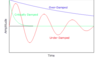21

22

23

24

Shown below is an example of a 'black body radiation curve' which shows the irradiance of all wavelengths of radiation emitted by an object.What is the relationship between the 'peak wavelength' emitted by an object and its temperature in kelvin?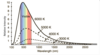25

26

27

28

Derive an expression for the kinetic energy of an object underging simple harmonic motion.29

30

The displacement of an object undergoing simple harmonic motion can be described by the relationshipsy = A sin ωtandy = A cos ωt(depending on initial conditions.)Sketch a displacement time graph for each of these situations.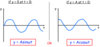31

32

33

34

35

36

37

38

39

40

41

42

43

44

45

The relationship shown below can be used to determine the wavelength of a laser going through a double slit.Define each of the quantities in the relationship.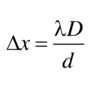46

The relationship shown below can be used to determine the wavelength of a laser going through a double slit.What effect would be seen on the interference pattern if a smaller wavelength of light was used?47

Plane polarised light can be produced by partial reflection from a glass surface as shown below.The reflected ray will be plane polarised if the angle of incidence is equal to the brewster angle (ip).What condition must be met to find the brewster angle, and produce plane polarised light?The angle between the reflected and refracted rays must be 90 degrees as shown below. This will produce a reflected ray which is plane polarised parallel to the surface of the glass.48

49

50

51

52

Shown below is an example of interference by division of amplitude- a lens is 'bloomed' with a non-reflective coating.Derive the expression d = λ/4n where 'd' is the thickness of coating required to give destructive interference.53

54

55

56

57

A stationary wave pattern is shown below.What is the wavelength of the wave?58

An experiment designed to show 'thin wedge' interference is set up as shown below:What is the relationship used to describe thin wedge interference, and what does each quantity represent?∆x = distance between bright fringes (m)λ = wavelength of light (m)l = length of 'wedge' (m)d = height of 'wedge' at end (m)59

What effect would having a thinner 'wedge' (d) have on the spacing between bright fringes (∆x) in the following experiment?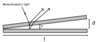A thinner wedge would make the spacing further apart.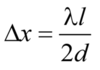60

61